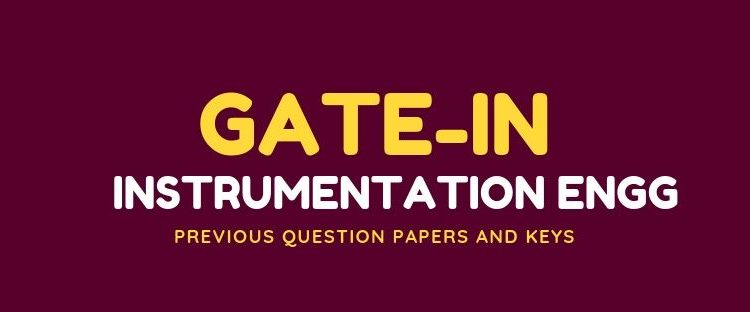GATE

# Past 12 Year Question Papers of GATE-IN Instrumentation Engg.

### GATE Instrumentation Engineering (IN) Previous 12 Years Question papers with answers.Graduate Aptitude Test in Engineering (GATE) is an examination conducted by the Indian Institute of Science (IISc), Bangalore along with seven Indian Institutes of Technology on behalf of MHRD and Government of India to test the understanding of the candidates in various undergraduate subjects related to Science. GATE Qualification is mandatory for seeking admission to ME, M. Arch and Doctoral programs in relevant branches of Science, in the institutions supported by the MHRD and other Government agencies. The score of GATE Examination is valid for Three Years from the announcement of the results and they are accepted by more than 900+ institutes across India.

A collection of previous years GATE IN Question papers with answers are provided below to give a better understanding about GATE Instrumentation Engineering upcoming examination.

For latest updates on GATE 2020, visit http://gate.iitm.ac.in/

The key points about GATE IN exam are:

• The exam is conducted ONLINE and is a duration of 3 hours.
• Questions are divided into three sections: General Aptitude, Engineering Mathematics, and Subject-specific part.
• Number of questions – 65 totaling to 100 marks
• Question type: Multiple Choice Questions (MCQs) and Numerical Answer Type (NAT) Questions of 1 mark and 2-marks respectively.
• Marking scheme: MCQs carry negative marks, while NAT questions don’t attract negative marking.

Here is a brief on the topics covered for GATE 2020 Instrumentation Engineering examination.

Syllabus of GATE IN Exam 2020:

• Section 1: Engineering Mathematics

Linear Algebra: Matrices and determinants, Eigen values and Eigen vectors.

Calculus: Partial derivatives; maxima and minima; Fourier series, Taylor series.

Vector Calculus: Gradient; divergence and curl; line; surface and volume integrals; Stokes, Gauss and Greens theorems.

Differential Equations: Cauchy’s and Euler’s equations; Laplace transforms; Partial Differential Equations – Laplace, Linear and non-linear first order Ordinary Differential Equations (ODE); Higher order linear ODEs with constant coefficients

Probability and Statistics: Poisson, normal and binomial distributions; correlation and regression analysis.

Numerical Methods: Solutions of linear and non-linear algebraic equations; numerical integration – trapezoidal and Simpson’s rule.

• Section 2: Electrical Circuits

Voltage and current sources: independent, dependent, ideal and practical. Kirchoffs laws,  Norton, maximum power transfer and reciprocity theorems. Peak-, average- and rms values of ac quantities; apparent-, active- and reactive powers.

• Section 3: Signals and Systems

Periodic, aperiodic and impulse signals, Discrete time system: impulse response, frequency response, pulse transfer function; DFT and FFT; basics of IIR and FIR filters.

• Section 4: Control Systems

Routh and Nyquist criteria, root loci, design of lead, lag and lead-lag compensators, state-space representation of systems; on-off, P, P-I, P-I-D, cascade, feedforward, and ratio controllers.

• Section 5: Analog Electronics

Characteristics and applications of diode, Zener diode, BJT and MOSFET; Characteristics of operational amplifiers; applications of opamps, Oscillators, signal generators, voltage controlled oscillators and phase locked loop.

• Section 6: Digital Electronics

Combinational logic circuits, minimization of Boolean functions. IC families, Characteristics of ADC and DAC; basics of number systems, 8-bit microprocessor and microcontroller.

• Section 7: Measurements

PMMC, MI and dynamometer type instruments; dc potentiometer; bridges for measurement of R, L and C, Q-meter. True rms meters, voltage and current scaling, instrument transformers, timer/counter, time, phase and frequency measurements, shielding and grounding.

• Section 8: Sensors and Industrial Instrumentation

Transducers for industrial instrumentation, liquid level, pH, conductivity and viscosity measurement.

• Section 9: Communication and Optical Instrumentation

Amplitude- and frequency modulation and demodulation; Shannon’s sampling theorem, pulse code modulation; optical sources and detectors: LED, laser, photo-diode, light dependent resistor and their characteristics; interferometer.

For the complete syllabus, click on GATE IN Syllabus 2020

Previous Year Question Papers along with the answer key are provided in the links below: